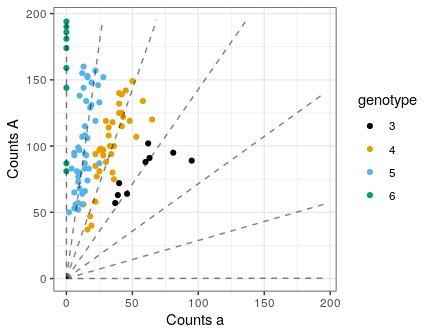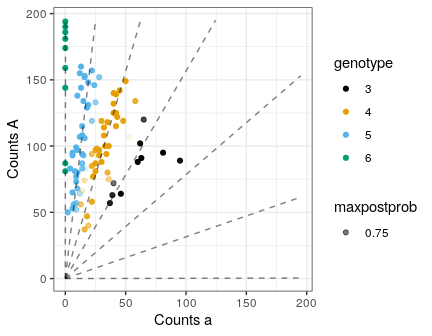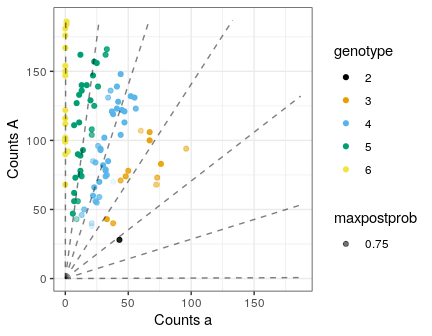# Abstract

We demonstrate how to simulate NGS data under various genotype distributions, then fit these data using flexdog.

# Analysis

Let’s suppose that we have 100 hexaploid individuals, with varying levels of read-depth.

set.seed(1)
library(updog)
nind    <- 100
ploidy  <- 6
sizevec <- round(stats::runif(n   = nind,
min = 50,
max = 200))

We can simulate their read-counts under various genotype distributions, allele biases, overdispersions, and sequencing error rates using the rgeno and rflexdog functions.

## F1 Population

Suppose these individuals are all siblings where the first parent has 4 copies of the reference allele and the second parent has 5 copies of the reference allele. Then the following code, using rgeno, will simulate the individuals’ genotypes.

true_geno <- rgeno(n      = nind,
ploidy = ploidy,
model  = "f1",
p1geno = 4,
p2geno = 5)

Once we have their genotypes, we can simulate their read-counts using rflexdog. Let’s suppose that there is a moderate level of allelic bias (0.7) and a small level of overdispersion (0.005). Generally, in the real data that I’ve seen, the bias will range between 0.5 and 2 and the overdispersion will range between 0 and 0.02, with only a few extremely overdispersed SNPs above 0.02.

refvec <- rflexdog(sizevec = sizevec,
geno    = true_geno,
ploidy  = ploidy,
seq     = 0.001,
bias    = 0.7,
od      = 0.005)

When we plot the data, it looks realistic

plot_geno(refvec  = refvec,
sizevec = sizevec,
ploidy  = ploidy,
bias    = 0.7,
seq     = 0.001,
geno    = true_geno)We can test flexdog on these data

fout <- flexdog(refvec  = refvec,
sizevec = sizevec,
ploidy  = ploidy,
model   = "f1")
#>          Fit: 1 of 5
#> Initial Bias: 0.3678794
#> Log-Likelihood: -363.9369
#> Keeping new fit.
#>
#>          Fit: 2 of 5
#> Initial Bias: 0.6065307
#> Log-Likelihood: -363.937
#> Keeping old fit.
#>
#>          Fit: 3 of 5
#> Initial Bias: 1
#> Log-Likelihood: -363.9369
#> Keeping new fit.
#>
#>          Fit: 4 of 5
#> Initial Bias: 1.648721
#> Log-Likelihood: -381.6123
#> Keeping old fit.
#>
#>          Fit: 5 of 5
#> Initial Bias: 2.718282
#> Log-Likelihood: -412.7397
#> Keeping old fit.
#>
#> Done!

flexdog gives us reasonable genotyping, and it accurately estimates the proportion of individuals mis-genotyped.

plot(fout)## Estimated proportion misgenotyped
fout$prop_mis #>  0.07011089 ## Actual proportion misgenotyped mean(fout$geno != true_geno)
#>  0.05

## HWE Population

Now run the same simulations assuming the individuals are in Hardy-Weinberg population with an allele frequency of 0.75.

true_geno <- rgeno(n      = nind,
ploidy = ploidy,
model  = "hw",
allele_freq = 0.75)
refvec <- rflexdog(sizevec = sizevec,
geno    = true_geno,
ploidy  = ploidy,
seq     = 0.001,
bias    = 0.7,
od      = 0.005)
fout <- flexdog(refvec  = refvec,
sizevec = sizevec,
ploidy  = ploidy,
model   = "hw")
#>          Fit: 1 of 5
#> Initial Bias: 0.3678794
#> Log-Likelihood: -377.9226
#> Keeping new fit.
#>
#>          Fit: 2 of 5
#> Initial Bias: 0.6065307
#> Log-Likelihood: -377.9226
#> Keeping old fit.
#>
#>          Fit: 3 of 5
#> Initial Bias: 1
#> Log-Likelihood: -377.9226
#> Keeping old fit.
#>
#>          Fit: 4 of 5
#> Initial Bias: 1.648721
#> Log-Likelihood: -377.9226
#> Keeping new fit.
#>
#>          Fit: 5 of 5
#> Initial Bias: 2.718282
#> Log-Likelihood: -377.9226
#> Keeping old fit.
#>
#> Done!
plot(fout)## Estimated proportion misgenotyped
fout$prop_mis #>  0.07625985 ## Actual proportion misgenotyped mean(fout$geno != true_geno)
#>  0.07

## Estimated allele frequency close to true allele frequency
fout$par$alpha
#>  0.7473264